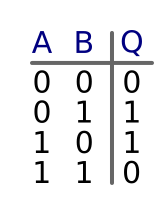# XOR gates

Martin McBride, 2017-01-04
Tags xor gate truth table
Categories logic logic gates

An Exclusive OR (XOR) gate has two inputs and one output. It is similar to an OR gate, except that the output obeys a slightly different rule.

The two inputs can each have values 0 or 1.

The state of the inputs controls the state of the output. The output value is 1 if either (but not both) inputs are 1, otherwise it is 0.

Another way to look at this is that the output will be 1 if the 2 inputs are different, but it will be 0 if both inputs are the same.

## Symbol

Here is the symbol for an XOR gate. The inputs are labelled A and B, the output is labelled Q.## Truth table

A truth table is a table which shows all possible combinations of inputs (A and B), and the resulting value of the output, Q. Here is the truth table for an XOR gate, as you can see the output is 1 if either (but not both) inputs are 1.## Interactive example

Here is an interactive XOR gate. Click on the inputs A and B to change their state, and see the output value Q.